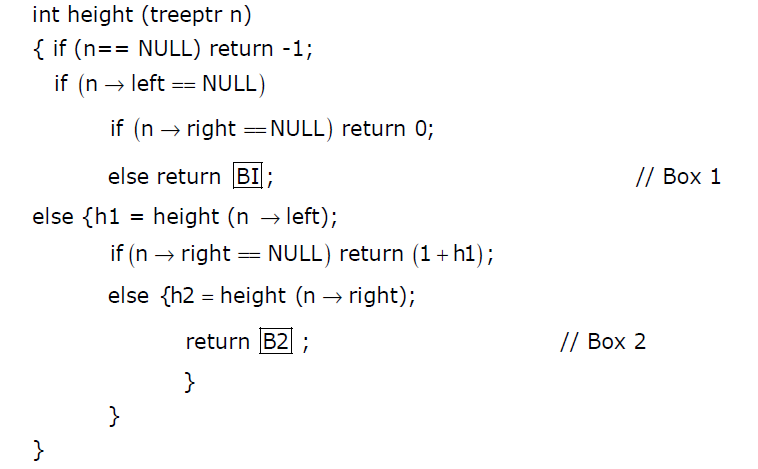Skip to content
Related Articles
Data Structures and Algorithms | Set 28
• Difficulty Level : Basic
• Last Updated : 11 Jun, 2021

Following questions have been asked in GATE 2012 exam.

1) Let w(n) and A(n) denote respectively, the worst case and average case running time of an algorithm executed on an input of size n. which of the following is ALWAYS TRUE?
(A) A(n) = Ω(W(n))
(B) A(n) = Θ(W(n))
(C) A(n) = O(W(n))
(D) A(n) = o(W(n))

Answer (C)
The worst case time complexity is always greater than or same as the average case time complexity.

2) The worst case running time to search for an element in a balanced in a binary search tree with n2^n elements is
(A) Θ(n log n)
(B) Θ(n2n
(C) Θ(n)
(D) Θ(log n)

Answer (C)
Time taken to search an element is Θ(h) where h is the height of Binary Search Tree (BST). The growth of height of a balanced BST is logarithmic in terms of number of nodes. So the worst case time to search an element would be Θ(Log(n*2^n)) which is Θ(Log(n) + Log(2^n)) Which is Θ(Log(n) + n) which can be written as Θ(n).

3) Assuming P != NP, which of the following is true ?
(A) NP-complete = NP
(B) NP-complete ∩ P = Φ
(C) NP-hard = NP
(D) P = NP-complete

Answer (B)
The answer is B (no NP-Complete problem can be solved in polynomial time). Because, if one NP-Complete problem can be solved in polynomial time, then all NP problems can solved in polynomial time. If that is the case, then NP and P set become same which contradicts the given condition.

4) The height of a tree is defined as the number of edges on the longest path in the tree. The function shown in the pseudocode below is invoked as height (root) to compute the height of a binary tree rooted at the tree pointer root.The appropriate expression for the two boxes B1 and B2 are
(A) B1 : (1 + height(n->right)), B2 : (1 + max(h1,h2))
(B) B1 : (height(n->right)), B2 : (1 + max(h1,h2))
(C) B1 : height(n->right), B2 : max(h1,h2)
(D) B1 : (1 + height(n->right)), B2 : max(h1,h2)

Answer (A)
The box B1 gets exected when left subtree of n is NULL and right sbtree is not NULL. In this case, height of n will be height of right subtree plus one.
The box B2 gets executed when both left and right sbtrees of n are not NULL. In this case, height of n will be max of heights of left and right sbtrees of n plus 1.

5) A list of n string, each of length n, is sorted into lexicographic order using the merge-sort algorithm. The worst case running time of this computation is
(A) O(n log n )
(B) O(n2 log n)
(C) O(n^2 + log n)
(D) O(n^2)

Answer (B)
The recurrence tree for merge sort will have height Logn. And O(n2) work will be done at each level of the recurrence tree (Each level involves n comparisons and a comparison takes O(n) time in worst case). So time complexity of this Merge Sort will be O(n2 log n).

Please see GATE Corner for all previous year paper/solutions/explanations, syllabus, important dates, notes, etc.

Please write comments if you find any of the answers/explanations incorrect, or you want to share more information about the topics discussed above.

Attention reader! Don’t stop learning now. Learn all GATE CS concepts with Free Live Classes on our youtube channel.

My Personal Notes arrow_drop_up